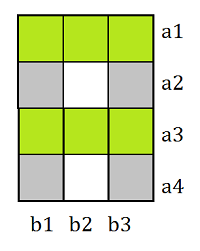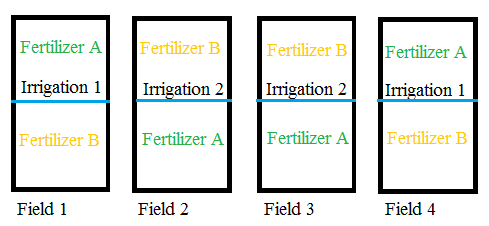# Strip-Plot / Split-Block Design

## What is a Strip-Plot Design?

Strip-plot designs (also called split-blocks) were originally used in wheat experiments (hence the word “plot” in the name). Today, they are also widely used in business and industry. Let’s say you had two factors A and B, each of which require large plot areas. Both factors have multiple levels a and b. For example, factor A could be irrigation; The levels (a) for irrigation could be: none, 1″ per day and 3″ per day. A complete factorial design—one which has each combination of each level—would require a*b large plots. If this isn’t practical (usually due to cost), a strip-plot design can be used as an alternative.

In a strip-plot design, the whole available area is divided into a horizontal strips and b vertical strips. One level of factor A is assigned to each row and one level of factor b is applied to each column. For example, if you had 3*4=12 factors, your plot would have 12 sections:A 3*4 split block design. The top left section would be assigned a1/b1 and the bottom right b3/a4.

## Split-Block vs. Split Plots

Although similar sounding, strip plots are not the same as split-plot designs. The main difference between split-block and split-plot experiments is the application of a second factor.

In a split-plot design, levels of a second factor are nested within a whole-plot factor. In the “usual” split-plot design, a mixture of hard to randomize (or hard-to-change) and easy-to-randomize (or easy-to-change) factors. The hard-to-change factors are implemented first, the fields are split in two, then the easier-to-change factors are implemented.In this split-plot design, Irrigation was implemented first followed by a split into two parts. Two fertilizers were randomized among the split plots.

In the split-block design, the “plots” are split horizontally and vertically according to how many levels are present in the experiment. In other words, the first, whole-plot factor is completely crossed with a second factor.

## References

Cheng, C. (2008). Complete factorial experiments in split-plots and strip-plots. Retrieved March 13, 2018 from: https://www.stat.berkeley.edu/~cheng/Lecture13.pdf
Federer, W. & King, F. (2007). Variations on Split Plot and Split Block Experiment Designs 1st Edition. Wiley-Interscience.
Visual Numerics (2003). Analyzing Statistically Designed Experiments. Retrieved March 13, 2018 from: https://www.roguewave.com/sites/rw/files/attachments/DOEWP.pdf

CITE THIS AS:
Stephanie Glen. "Strip-Plot / Split-Block Design" From StatisticsHowTo.com: Elementary Statistics for the rest of us! https://www.statisticshowto.com/strip-plot-block-design/